FIND

What you want a star-finder to do is find stars. Of course, it can't really do this - the most you can hope for is that it will identify most of the things in a digital image which look like stars. And the program really should consider how each object looks, because you can't just say that every group of pixels which is brighter than average is a star. In many CCD frames, especially those of star clusters or nearby galaxies, the diffuse sky brightness is different in different parts of the image. Any fixed brightness threshold which you would set might be just a little bit above the mean sky level in one part of the image, so that all sorts of noise spikes get picked up and identified as stars, while in some other part of the image the threshold is far above the local sky level, so that many faint stars are missed. What you really want to do is to search for little brightness peaks which stick up above the local sky brightness (whatever that happens to be) and which have approximately the correct diameter and profile for a star image in a frame of whatever sampling interval and seeing conditions you have. DAOPHOT does this in a crude way, by means of linear least squares.

The program asks the user to specify the full-width at half-maximum expected for stars in that frame, which is just the seeing diameter. (Precise knowledge of the actual seeing for the frame isn't really essential - it's usually adequate to divide the site's typical seeing, in arcseconds, by the scale of the telescope, in arcseconds per pixel, to get a good enough guess at the FWHM, in pixels. Although it is true that the seeing does vary from one frame to another in the course of a given night or observing run, it's unusual for it to vary by more than a factor of two or so. If necessary, for any given frame it's easy enough to estimate the FWHM by dumping or plotting the data in and around some bright star.) Once given a numerical value for the FWHM, DAOPHOT's FIND routine then assumes that the stellar profile is a circular Gaussian function with that full-width at half-maximum, and just goes through the entire picture fitting Gaussian profiles to a small region around every single pixel (excluding a narrow border around the frame): for each pixel (i0, j0) it performs the following fitwhere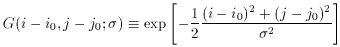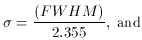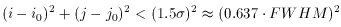Notice that this is linear least squares so, in the notation of my first lecture, FIND can compute N,x and,x2 ahead of time, and only needs to calculatey andxy afresh for each point. Then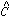andfall out directly. The value of(i0,j0), where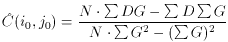(look familiar?) observed for the region around each (i0, j0) tells FIND, if there were a star centered at this (i0, j0), how bright it would be above the local background., which is the estimate of the local sky brightness, is not needed so FIND doesn't bother to compute it. In this way FIND builds up a new image,(i0, j0), which has the local background, and any simple gradients in the background, completely removed. (Since the fitting region around the point is symmetric, a gradient's positive contribution to the sums on one side is canceled by the equal negative contribution on the other.) It is then simply a matter of picking out those pixels (a) where the value ofexperiences a local maximum (simply: any pixel where the value ofis larger than in any other pixel within some radius), in order to choose only the one pixel which is closest to the center of any given star; and (b) whereis larger than some user-specified multiple of(C), to eliminate random noise peaks.

There are a couple of little refinements that DAOPHOT's FIND adds to this essentially trivial concept. First, the user is allowed to specify two "bad-pixel" thresholds: the algorithm discards as "bad" any pixel whose brightness value is below the first threshold (which the user should put, say, 7below the frame's mean sky level) or above the second (which should be the point at which the CCD response becomes nonlinear due to incipient saturation). To "discard" a pixel simply means that that pixel is decremented from the sums in the equation for. Thus, image flaws and bright cosmic-ray events are not carried over into the derived image(i0, j0), at a very small cost in extra computing time. Second, after statistically significant local maxima inhave been found, two intensity moments are computed from the corresponding pixels in the original image, D. These represent the sharpness (brightness in the central pixel of the peak as compared to the surrounding pixels) and roundness (second derivative of intensity with respect to the y direction, as compared to second derivative with respect to x). By rejecting oddballs on the basis of these criteria, FIND can go a long way toward eliminating cosmic rays, charge-overflow columns, and other image flaws which weren't extreme enough to be eliminated by the bad-data thresholds. Some background galaxies are also eliminated by these limits, which could be an advantage or a disadvantage depending on what you're doing. Finally, the FIND algorithm carries out precisely one iteration's worth of nonlinear least squares on all detections which have survived the various tests, to improve the estimate of the centroid position to better than the nearest pixel. These typically come out precise to of order 0.2 pixels.